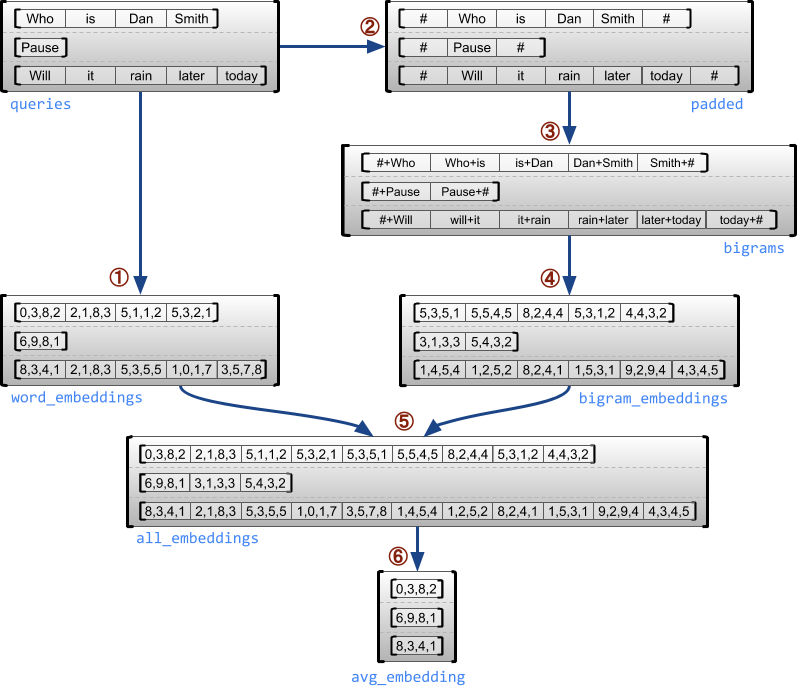Introducing Ragged Tensors
December 12, 2018
Posted by Laurence Moroney

In many scenarios, data doesn’t come evenly divided into uniformly-shaped arrays that can be loaded into tensors. A classic case is in training and processing text. For example, if you look at the Text Classification tutorial that uses the IMDB dataset, you’ll see a major part of your data preparation is in shaping your data to a normalized size. In that case, every review needs to be 256 words long. If it is longer, it is truncated, and if it is shorter, it is padded with 0 values until it reaches the desired length.

Ragged tensors are designed to ease this problem. They are the TensorFlow equivalent of nested variable-length lists. They make it easy to store and process data with non-uniform shapes, such as:
• Feature columns for variable-length features, such as the set of actors in a movie.
• Batches of variable-length sequential inputs, such as sentences or video clips.
• Hierarchical inputs, such as text documents that are subdivided into sections, paragraphs, sentences, and words.
• Individual fields in structured inputs, such as protocol buffers.
So, for example, consider a speech like this, where the length may change wildly on each line:
``````speech = tf.ragged.constant(
[['All', 'the', 'world', 'is', 'a', 'stage'],
['And', 'all', 'the', 'men', 'and', 'women', 'merely', 'players'],
['They', 'have', 'their', 'exits', 'and', 'their', 'entrances']])``````
When printing this out, we can see that it is created from a list of lists, with each being of variable length:
``<tf.RaggedTensor [['All', 'the', 'world', 'is', 'a', 'stage'], ['And', 'all', 'the', 'men', 'and', 'women', 'merely', 'players'],  ['They', 'have', 'their', 'exits', 'and', 'their', 'entrances']]>``
Most operations that you’d expect to be supported with normal tensors are available to ragged tensors too, so, for example, Python style indexing to access slices of the tensor work as expected:
``````>>print(speech)
tf.Tensor(['All', 'the', 'world', 'is', 'a', 'stage'], shape=(6,), dtype=string)``````
The `tf.ragged` package also defines a number of operations that are specific to ragged tensors. For example, the `tf.ragged.map_flat_values` operation can be used to efficiently transform the individual values in a ragged tensor, while keeping its shape the same:
``````> print tf.ragged.map_flat_values(tf.strings.regex_replace,speech, pattern="([aeiouAEIOU])", rewrite=r"{\1}")
``````

### Ragged and Sparse

It’s important to note that a ragged tensor is not the same as a sparse tensor, but rather as a dense tensor with an irregular shape. The key difference here is that a ragged tensor keeps track of where each row begins and ends, whereas a sparse one needs to keep track of the coordinates of each individual item. You can see the impact of this by exploring a concatenation of sparse and ragged tensors. Concatenating sparse tensors is equivalent to concatenating the corresponding dense tensors, as illustrated by the following example (where Ø indicates missing values):But when concatenating ragged tensors, each individual row is joined, to form a single row with the combined length:### Using Ragged Tensors

The following example shows ragged tensors being used to construct and combine embeddings of single words (unigrams) and word pairs (bigrams) for a variable-length list of words making up a phrase. You can also try this code for yourself in the Ragged Tensor Guide.
``````import math
import tensorflow as tf
tf.enable_eager_execution()
# Set up the embeddingss
num_buckets = 1024
embedding_size = 16
embedding_table =
tf.Variable(
tf.truncated_normal([num_buckets, embedding_size],
stddev=1.0 / math.sqrt(embedding_size)),
name="embedding_table")
# Input tensor.
queries = tf.ragged.constant([
['Who', 'is', 'Dan', 'Smith']
['Pause'],
['Will', 'it', 'rain', 'later', 'today']])
# Look up embedding for each word.  map_flat_values applies an operation to each value in a RaggedTensor.
word_buckets = tf.strings.to_hash_bucket_fast(queries, num_buckets)
word_embeddings = tf.ragged.map_flat_values(
tf.nn.embedding_lookup, embedding_table, word_buckets)  # ①
# Add markers to the beginning and end of each sentence.
marker = tf.fill([queries.nrows()), 1], '#')
padded = tf.concat([marker, queries, marker], axis=1)           # ②
# Build word bigrams & look up embeddings.
bigrams = tf.string_join(
bigram_buckets =
tf.strings.to_hash_bucket_fast(bigrams, num_buckets)
bigram_embeddings = tf.ragged.map_flat_values(
tf.nn.embedding_lookup, embedding_table, bigram_buckets)   # ④
# Find the average embedding for each sentence
all_embeddings =
tf.concat([word_embeddings, bigram_embeddings], axis=1)    # ⑤
avg_embedding = tf.reduce_mean(all_embeddings, axis=1)         # ⑥
print(word_embeddings)
print(bigram_embeddings)
print(all_embeddings)
print(avg_embedding)``````
This is illustrated in the following diagram. Note that the numbers are for illustrative purposes only. For the real values in the embedding, check out the values output at the end of the code block.### Conclusion

As you can see, ragged tensors are very useful for a variety of scenarios, preventing you from needing to create equally sized and ranked lists. Consider using them when storing and processing data with non-uniform shapes such as variable-length features like the cast of a movie; batches of variable-length sequential inputs such as sentences; hierarchical data structures, such as documents that are subdivided into sections, paragraphs, sentences and words or individual fields in structured inputs such as protocol buffers. For these use cases, ragged tensors are more efficient than a padded `tf.Tensor`, since no time or space is wasted on the padding values; and are more flexible and convenient than using a `tf.SparseTensor`, since they support a wide variety of operations, with the correct semantics for variable-length lists.

Currently, ragged tensors are supported by the low-level TensorFlow APIs; but in the coming months, we will be adding support for processing RaggedTensors throughout the Tensorflow stack, including Keras layers and TFX.

This barely touches the surface of ragged tensors, and you can learn more about them on the Ragged Tensor Guide. For more documentation on ragged tensors, see the tf.ragged package documentation on TensorFlow.org.
Next postPosted by Laurence Moroney

In many scenarios, data doesn’t come evenly divided into uniformly-shaped arrays that can be loaded into tensors. A classic case is in training and processing text. For example, if you look at the Text Classification tutorial that uses the IMDB dataset, you’ll see a major part of your data preparation is in shaping your data to a normalized size. In that case, every rev…